# exp-6 potential

(Redirected from Exp6 potential)

The exp-6 potential (or Exp-Six potential) is a modified form of the Buckingham potential and is given by (Eq. 1 in ):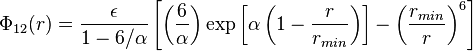$\Phi_{12}(r) = \frac{\epsilon}{1-6/\alpha} \left[ \left( \frac{6}{\alpha} \right) \exp \left[ \alpha \left( 1-\frac{r}{r_{min}} \right) \right] - \left( \frac{r_{min}}{r}\right)^6 \right]$

where

•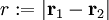$r := |\mathbf{r}_1 - \mathbf{r}_2|$
•$\Phi_{12}(r)$ is the intermolecular pair potential between two particles or sites
•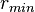$r_{min}$ is the value of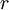$r$ at which$\Phi_{12}(r)$ is a minimum.
•$\epsilon$ is the well depth (energy)
•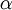$\alpha$ is the "steepness" of the repulsive energy

## Melting point

An approximate method to locate the melting point is given in . See also .